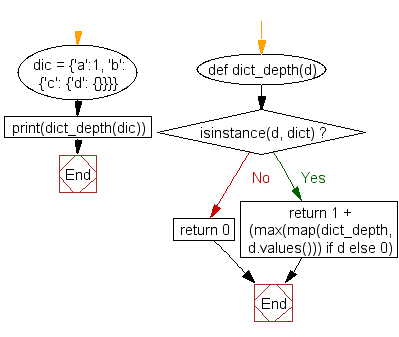﻿ Python: Get the depth of a dictionary - w3resource# Python: Get the depth of a dictionary

## Python Dictionary: Exercise - 54 with Solution

Write a Python program to get the depth of a dictionary.

Sample Solution:-

Python Code:

``````def dict_depth(d):
if isinstance(d, dict):
return 1 + (max(map(dict_depth, d.values())) if d else 0)
return 0
dic = {'a':1, 'b': {'c': {'d': {}}}}
print(dict_depth(dic))
```
```

Sample Output:

```4
```

Flowchart:## Visualize Python code execution:

The following tool visualize what the computer is doing step-by-step as it executes the said program:

Python Code Editor:

Have another way to solve this solution? Contribute your code (and comments) through Disqus.

What is the difficulty level of this exercise?

Test your Programming skills with w3resource's quiz.

﻿

## Python: Tips of the Day

Invokes the provided function after ms milliseconds:

Example:

```from time import sleep

def tips_delay(fn, ms, *args):
sleep(ms / 1000)
return fn(*args)
print(tips_delay(
lambda x: print(x),
1000,
'w3r'
))
```

Output:

```w3r
None
```